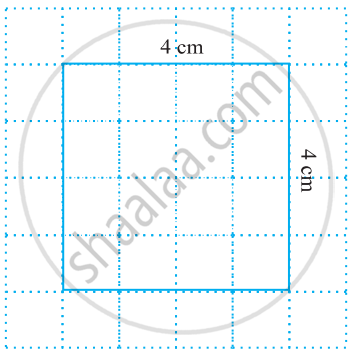# Area of Square

#### formula

Area of a square = side × side = (Side)2

# Area of a square:The area of the square is equal to the length of one side squared.

Area of a square = side × side = (Side)2 = a2, where a is the length of each side.

Let us now consider a square of side 4 cm

It covers 16 squares i.e. the area of the square

= 4 × 4 sq. cm.

= 16 sq. cm.

We find that in each case,

Area of the square = side × side

#### Example

Find the area of a square plot of side 8 m.

Side of the square = 8 m

Area of the square= side × side

= 8 m × 8 m

= 64 sq m.

#### Example

Bob wants to cover the floor of a room 3 m wide and 4 m long by squared tiles. If each square tile is of side 0.5 m, then find the number of tiles required to cover the floor of the room.

Total area of tiles must be equal to the area of the floor of the room.
Length of the room = 4 m
Breadth of the room = 3 m

Area of the floor = length × breadth = 4 m × 3 m = 12 sq m

Area of one square tile = side × side = 0.5 m × 0.5 m = 0.25 sq m

Number of tiles required = "Area of the floor"/"Area of one tile" = 12/0.25 = 1200/25 = 48 tiles.

If you would like to contribute notes or other learning material, please submit them using the button below.

### Shaalaa.com

Area Of A Square [00:07:07]
S
0%